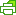# What is the MAC and how is it calculated?

The Moving Average Cost (MAC) is how TradeGecko determines the cost of a product at any point after a Purchase Order, or Stock Adjustment has been made, or when a Sales Order has been shipped.The MAC is recalculated by taking the MAC before the Purchase Order and the cost price of the Purchase Order and then averaging them out. The same method of recalculation applies for stock adjustments.

The result of the recalculation will become the current MAC.

For stock adjustments, the MAC only changes when the cost of the stock adjustment add or deducts from the current MAC, thus altering it.

Note
The MAC is calculated based on base currency. Therefore, when you have a Purchase Order in a different currency, TradeGecko will convert it to the base currency and calculate it for you.

Here’s an example of how MAC is calculated:

You have 10 wicker baskets in stock on April 1, at a cost per unit of \$5. You sell 5 units on April 5, and record a charge to the cost of goods sold of \$25, which is calculated from 5 units x \$5 per unit. This means there are now 5 units remaining in stock, at a cost per unit of \$5 and a total cost of \$25.

You then purchase 50 additional wicker baskets on April 10 for \$6 each (total purchase of \$300). The moving average cost is now \$5.90, which is calculated as a total cost of \$325 divided by the 55 units still on hand.

On April 12, you sell 10 units and record a charge to the cost of goods sold of \$59, which is calculated from 10 units x \$59 per unit. This means there are now 45 units remaining in stock, at a cost per unit of \$5.90 and a total cost of \$265.50.

Therefore, you began the month with a \$50 beginning balance of 10 wicker baskets at a cost of \$5 each, sold 5 units at a cost of \$5 on April 5, revises the moving average cost to \$5.90 after a purchase on April 10; sells 10 units at a cost of \$5.90 on April 12. You can see that the cost per unit changes following an inventory purchase, but not after an inventory sale.PrintPDF
Have more questions? Submit a request